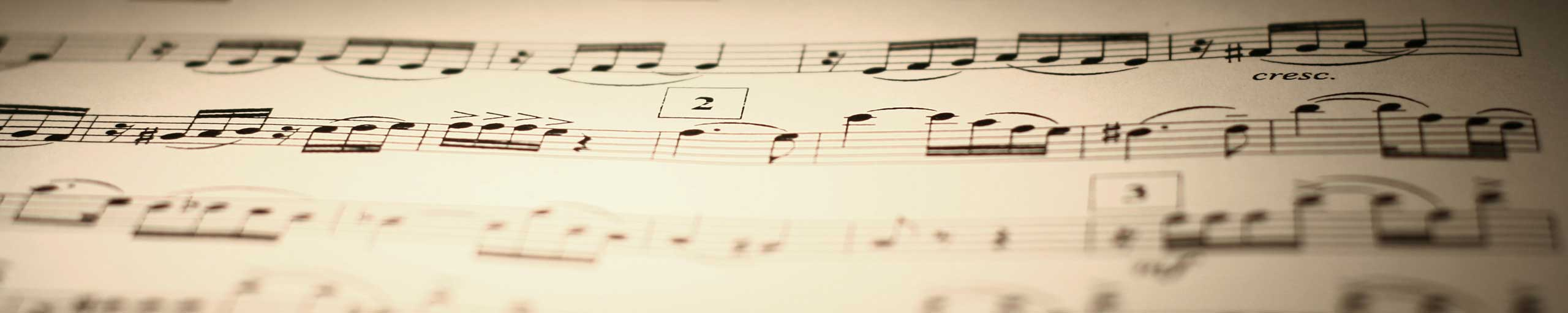# Solve Second Order Differential Equations - part 2

Solve second order differential equations with auxiliary equation having 2 equal real solutions. Examples with detailed solutions are included.

 Given the auxiliary equation of a second order differential equation k2 + b k + c = 0 Show that if b 2 - 4 c = 0, in which case the above equation gives two equal real solution, y = x ekx is also a general solution to the second order differential equation d2y / dx2 + b dy / dx + c y = 0. If y = x ekx, then y' = ekx + k x ekx and y" = 2 k ekx + x k2ekx. Substitute y, y' and y" obtained above into the differential equation, we obtain 2 k ekx + x k2ekx + b (ekx + k x ekx) + c(x ekx) = 0 If b 2 - 4 c = 0, the auxiliary equation has two real equal solutions given by k = - b / 2. Substitute k by - b / 2 in the above equation, collect like terms and simplify to obtain ekx[ - b2 / 4 + c] = 0. Since b 2 - 4 c = 0, [ - b2 / 4 + c] is also equal to zero and hence the suggested solution satisfies the differential equation given above. In case the auxiliary equation gives two equal real solutions, the solution to the differential equation is given by y = A e kx + B x ekx Using examples, It will be shown how one can solve equation. Example 1: Solve the second order differential equation given by y" + 2 y' + y = 0 Solution to Example 1 The auxiliary equation is given by k2 + 2 k + 1 = 0 Factor the above quadratic equation and solve for k (k + 1)2 = 0 k = - 1 , two equal real solutions. The general solution to the given differential equation is given by y = A e k x + B x e k x = A e - x + B x e- x where A and B are constants. Example 2: Solve the second order differential equation given by y" - 4 y' + 4 y = 0 with the initial conditions y(0) = 4 and y'(0) = 0 Solution to Example 2 The auxiliary equation is given by k2 - 4 k + 4 = 0 The above quadratic equation has two equal real solutions k = 2 The general solution is given by y = A e2 x + B x e2 x where A and B are constants and are evaluated using the initial conditions. y(0) = 4 gives y(0) = A e0 + 0 = A = 4 y'(0) = 0 gives y'(0) = 2 A e0 + B ( e0 + 2 (0) e0) = 2A + B = 0 Solve the system of equations A = 4 and 2 A + B = 0 to obtain A = 4 and B = -8 The solution may be written as y = 4 e2 x - 8 x e2 x More references on Differential Equations Differential Equations - Runge Kutta Method